# Min and max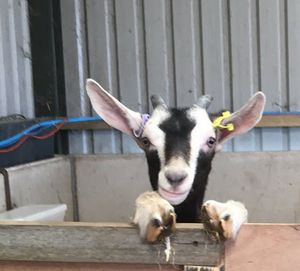Cutie!
Photo: Holly Vickery

Often you want to find the smallest (minimum) and largest (maximum) values in a data set. For example, the goat with the smallest weight, and the goat with the largest weight. This lesson is about how you do that.

We'll use this small data set...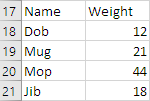... but the method will work for a data set of any size.

### Heaviest goat

Let's start by finding the heaviest goat. Assume for now there are no data errors. You already know how to do validation on a `Range`.

Here's the worksheet we'll use.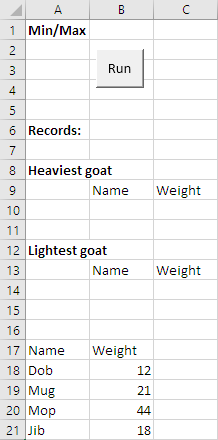Let's write code to fill in the record count, and heaviest goat cells.

Here's the general approach:

• Set up the range
• For each record
•     Get weight for current record
•     If weight > highest weight so far:
•         highest weight so far = weight

So, we make a new variable, to hold the highest weight. Work through the records. For each one, compare the weight in the record with the highest one we've found. If the current record's weight is greater, we have a new highest weight.

Let's make the `Range` and the loop.

1. 'Set up the Range.
2. Set rGoatData = Range(Cells(18, 1), Cells(18, 1).End(xlToRight).End(xlDown))
3. sNumberOfRecords = rGoatData.Rows.Count
4. 'Loop over records.
5. For sCurrentRecordNumber = 1 To sNumberOfRecords
6.     tName = rGoatData.Cells(sCurrentRecordNumber, 1)
7.     sWeight = rGoatData.Cells(sCurrentRecordNumber, 2)
8.
9.     CODE TO FIND HEAVIEST
10.
11. Next sCurrentRecordNumber
12. 'Output
13. Cells(6, 2) = sNumberOfRecords
14. Cells(10, 2) = tNameHeaviest
15. Cells(10, 3) = sMaximumWeight

Check out the last two lines. To output the name of the heaviest goat, we'll need a variable with the name in it. So that's one thing our processing code will have to find.

To output the heaviest weight, we'll need a variable for that. The processing code will work out the variable's value.

Here's code that will do it. Some `Dim`s are included, for extra things we need.

1. Dim sMaximumWeight As Single
2. Dim tNameHeaviest As String
3. ...
4. 'Set up the Range.
5. Set rGoatData = Range(Cells(18, 1), Cells(18, 1).End(xlToRight).End(xlDown))
6. sNumberOfRecords = rGoatData.Rows.Count
7. 'Loop over records.
8. For sCurrentRecordNumber = 1 To sNumberOfRecords
9.     tName = rGoatData.Cells(sCurrentRecordNumber, 1)
10.     sWeight = rGoatData.Cells(sCurrentRecordNumber, 2)
11.     'Test for max.
12.     If sWeight > sMaximumWeight Then
13.         sMaximumWeight = sWeight
14.         tNameHeaviest = tName
15.     End If
16. Next sCurrentRecordNumber
17. 'Output
18. Cells(6, 2) = sNumberOfRecords
19. Cells(10, 2) = tNameHeaviest
20. Cells(10, 3) = sMaximumWeight

Go through the records, one at a time. `sMaximumWeight` holds the maximum weight we've found so far.

Each time we grab a record, compare its weight to the `sMaximumWeight`. If the record's weight is higher, remember the new maximum, and the name of that goat.

When we get to the end of the data set, `sMaximumWeight` will have the highest weight, and `tNameHeaviest` will have the name of that goat.

Let's give it a try.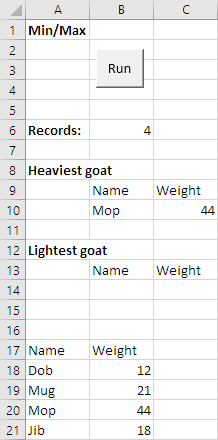Yay! It worked!

### Lightest goat

Let's do the same thing for the lightest goat.

1. Dim sMaximumWeight As Single
2. Dim tNameHeaviest As String
3. Dim sMinimumWeight As Single
4. Dim tNameLightest As String
5. ...
6. 'Set up the Range.
7. Set rGoatData = Range(Cells(18, 1), Cells(18, 1).End(xlToRight).End(xlDown))
8. sNumberOfRecords = rGoatData.Rows.Count
9. 'Loop over records.
10. For sCurrentRecordNumber = 1 To sNumberOfRecords
11.     tName = rGoatData.Cells(sCurrentRecordNumber, 1)
12.     sWeight = rGoatData.Cells(sCurrentRecordNumber, 2)
13.     'Test for max.
14.     If sWeight > sMaximumWeight Then
15.         sMaximumWeight = sWeight
16.         tNameHeaviest = tName
17.     End If
18.     'Test for min.
19.     If sWeight < sMinimumWeight Then
20.         sMinimumWeight = sWeight
21.         tNameLightest = tName
22.     End If
23. Next sCurrentRecordNumber
24. 'Output
25. Cells(6, 2) = sNumberOfRecords
26. Cells(10, 2) = tNameHeaviest
27. Cells(10, 3) = sMaximumWeight
28. Cells(14, 2) = tNameLightest
29. Cells(14, 3) = sMinimumWeight

Same code, but with a less than in the `If`, rather than a greater than.

Let's try it.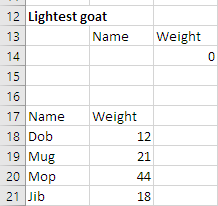Wait, what!? The lightest goat is weightless?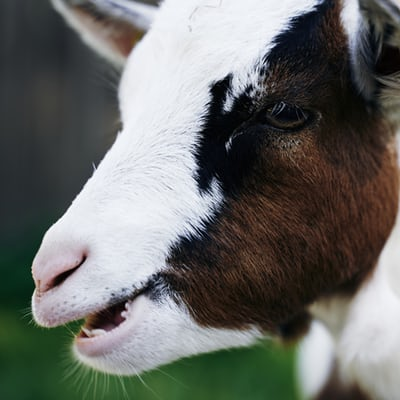That doesn't look right.

The lightest is supposed to be 12.

Hey, Georgina. Can you work out the problem?Georgina

I'll try. Let's see... I'll add a breakpoint in the processing loop. Let's see what happens.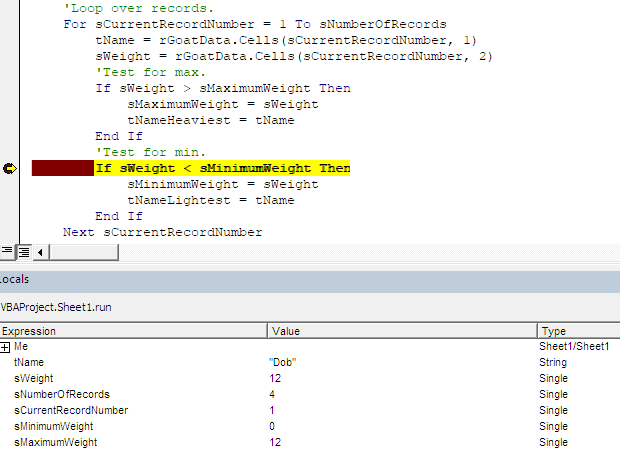The first time through the loop, `sCurrentRecord` is 1. The weight for that record, in `sWeight`, is 12.Georgina

OK, I see the problem. The `If` is comparing the goat weight (that's 12) with zero.

• If sWeight 12 < sMinimumWeight 0 Then

All of the weights are more than zero, so `sMinimumWeight` is never changed.Ray

Why is `sMinimumWeight` zero?

Wait..., never mind. It starts out at zero. That's what all variables start out as. Well, all `Single`s, anyway.

Right. The problem is that `sMinimumWeight` starts out at 0, which is smaller than the actual smallest value in the data, so it never gets changed by the `If` statement.

To fix it, let's initialize `sMinimumWeight` to a very large value. We know no goats weight a million kilos, so we could do something like this:

• sMinimumWeight = 1000000
• 'Loop over records.
• For sCurrentRecordNumber = 1 To sNumberOfRecords
•     tName = rGoatData.Cells(sCurrentRecordNumber, 1)
•     sWeight = rGoatData.Cells(sCurrentRecordNumber, 2)
•     ...
•     'Test for min.
•     If sWeight < sMinimumWeight Then
•         sMinimumWeight = sWeight
•         tNameLightest = tName
•     End If
• Next sCurrentRecordNumber

All of the records will have weights less than 1,000,000 kilos. The first time through the loop, the weight in the first record will be less than 1,000,000, so it will become the new minimum at that point.

So we should get the smallest. Let's try it.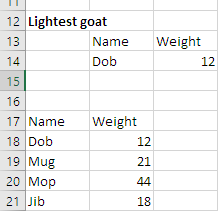Yay!

We can do a little better. Instead of 1,000,000, we could set `sMinimumWeight` to the largest number VBA can put in a `Single`.

• Dim sMinimumWeight as Single
• ...
• sMinimumWeight = 3.4028235E+38
• For sCurrentRecord = 1 To sNumberRecords

`3.4028235E+38` is 34,028,235,000,000,000,000,000,000,000,000,000,000. The `E` means "times ten to the".Should we make the same change to the maximum code? Just in case.

Oh, right! Yes, we should. `sMaximumWeight` starts off at zero, and all of the goat weights will be more than that.

But if we were dealing with, say, temperature data for different places, it's possible that all of the temperatures would be negative. Then we'd get zero for the maximum, rather than the right value.

So let's initialize `sMaximumWeight` to the smallest possible value. Every real value will be bigger than that.

• Dim sMaximumWeight as Single
• ...
• sMaximumWeight = -3.4028235E+38
• For sCurrentRecord = 1 To sNumberRecordsEthan

OK, we want the lightest goat, and the heaviest goat. Would you have two separate loops, or put everything into one loop?

I'd put everything into one loop. Like this:

• 'Set up the Range.
• Set rGoatData = Range(Cells(18, 1), Cells(18, 1).End(xlToRight).End(xlDown))
• sNumberOfRecords = rGoatData.Rows.Count
• 'Initialize min weight to highest number possible for a Single.
• sMinimumWeight = 3.4028235E+38
• 'Initialize max weight to most negative value possible for a Single.
• sMaximumWeight = -3.4028235E+38
• 'Loop over records.
• For sCurrentRecordNumber = 1 To sNumberOfRecords
•     tName = rGoatData.Cells(sCurrentRecordNumber, 1)
•     sWeight = rGoatData.Cells(sCurrentRecordNumber, 2)
•     'Test for max.
•     If sWeight > sMaximumWeight Then
•         sMaximumWeight = sWeight
•         tNameHeaviest = tName
•     End If
•     'Test for min.
•     If sWeight < sMinimumWeight Then
•         sMinimumWeight = sWeight
•         tNameLightest = tName
•     End If
• Next sCurrentRecordNumber
• 'Output
• Cells(6, 2) = sNumberOfRecords
• Cells(10, 2) = tNameHeaviest
• Cells(10, 3) = sMaximumWeight
• Cells(14, 2) = tNameLightest
• Cells(14, 3) = sMinimumWeight

### A new pattern

Now we have a pattern for min/max. You can use it whenevs.

Pattern

Loop across some records. Put an `If` in the loop, comparing the current value with the smallest/largest so far.

### Exercise

Exercise

Dancing with the goats

Download the starting workbook. It has data on scores from an episode of the new hit TV show, "Dancing with the Goats."

It starts like this: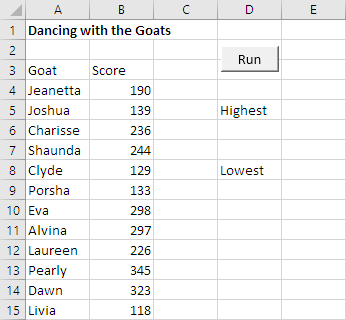Click the button, and see the goats with the highest and lowest scores.Write the code so it would still work if the number of records changed.